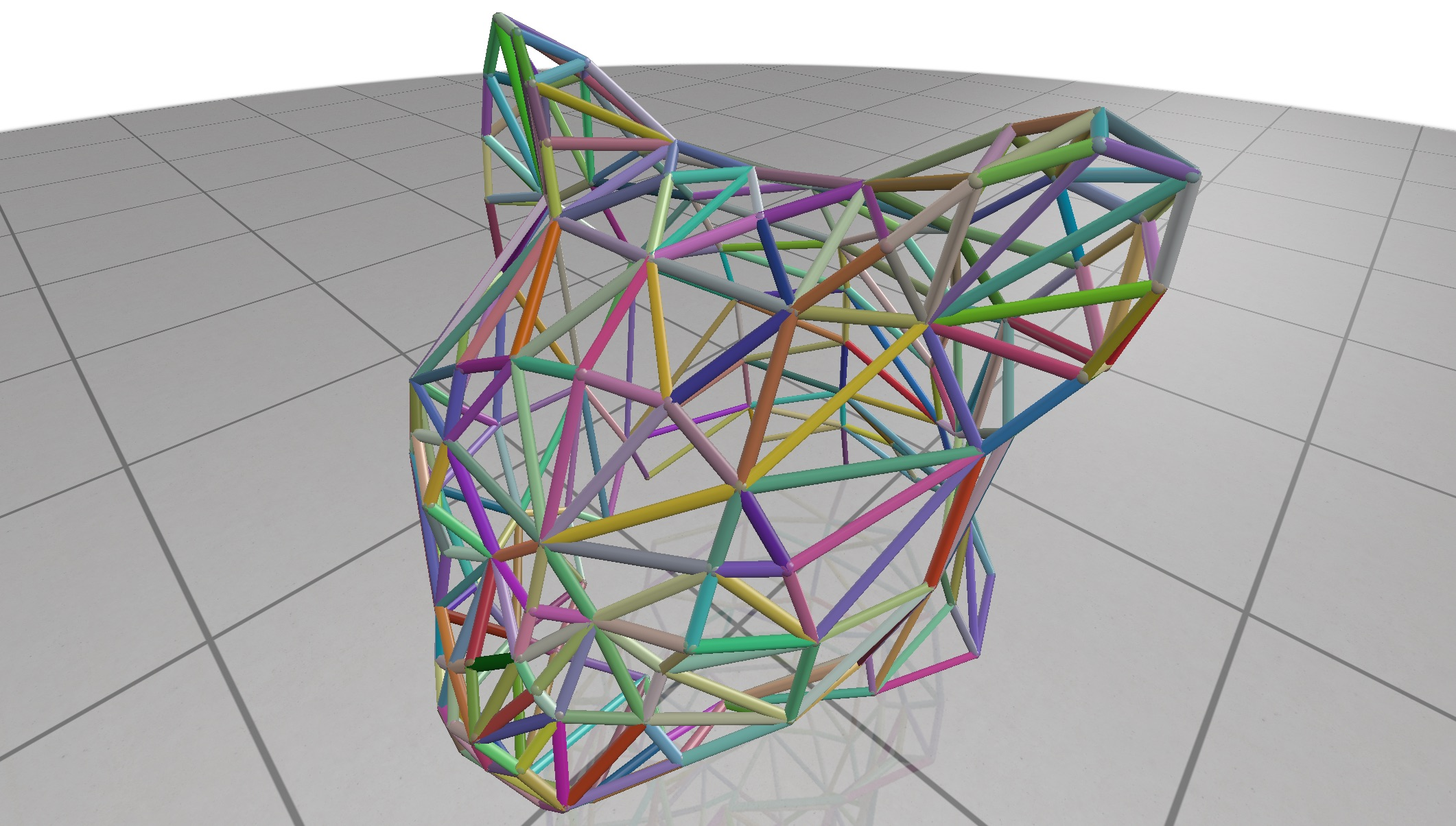# Color Quantities

Visualize color rgb-valued data at the nodes or edges of a curve network.Example:

#include "polyscope/curve_network.h"

std::vector<std::array<double, 3>> randColor(edges.size());
for (size_t i = 0; i < edges.size(); i++) {
randColor[i] = {{polyscope::randomUnit(), polyscope::randomUnit(), polyscope::randomUnit()}};
}

// visualize


CurveNetwork::addNodeColorQuantity(std::string name, const T& values)

Add a color quantity to the nodes of the curve network

• values is the array of colors at nodes. The type should be adaptable to a 3-vector array of floats. The length should be the number of nodes in the curve network.

RGB values are interpreted in the range [0,1].

CurveNetwork::addEdgeColorQuantity(std::string name, const T& values)

Add a color quantity to the edges of the curve network

• values is the array of colors at edges. The type should be adaptable to a 3-vector array of floats. The length should be the number of edges in the curve network.

RGB values are interpreted in the range [0,1].